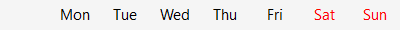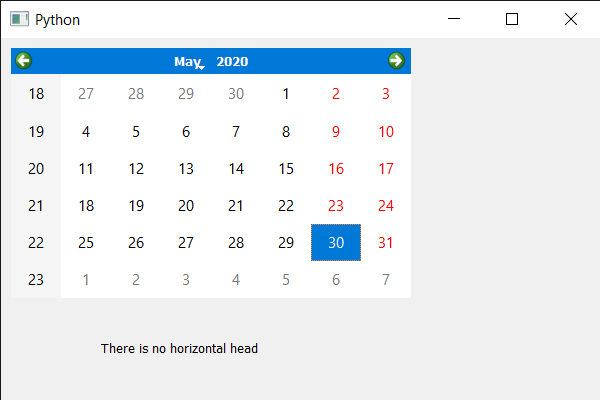# PyQt5 QCalendarWidget – Checking if there is horizontal header or not

• Last Updated : 25 May, 2022

In this article, we will see how we check if the QCalendarWidget has the horizontal header or not. Horizontal header is the place in QCalendarWidget which shows the days name by default short form of days are shown for example Monday is displayed as Mon, below is the horizontal header representation.Horizontal can be removed using overriding the structure of the QCalendarWidget or by setting header format value to 0, therefore in order to check if there is header or not we have to get the value of the header and have to compare with the 0 value to know if there is header or not.

Below is the implementation

## Python3

 `# importing libraries``from` `PyQt5.QtWidgets ``import` `*``from` `PyQt5 ``import` `QtCore, QtGui``from` `PyQt5.QtGui ``import` `*``from` `PyQt5.QtCore ``import` `*``import` `sys`  `class` `Window(QMainWindow):` `    ``def` `__init__(``self``):``        ``super``().__init__()` `        ``# setting title``        ``self``.setWindowTitle(``"Python"``)` `        ``# setting geometry``        ``self``.setGeometry(``100``, ``100``, ``600``, ``400``)` `        ``# calling method``        ``self``.UiComponents()` `        ``# showing all the widgets``        ``self``.show()` `    ``# method for components``    ``def` `UiComponents(``self``):` `        ``# creating a QCalendarWidget object``        ``calendar ``=` `QCalendarWidget(``self``)` `        ``# setting geometry to the calendar``        ``calendar.setGeometry(``10``, ``10``, ``400``, ``250``)` `        ``# removing horizontal header``        ``calendar.setHorizontalHeaderFormat(``0``)` `        ``# creating a label``        ``label ``=` `QLabel(``self``)` `        ``# setting geometry to the label``        ``label.setGeometry(``100``, ``280``, ``250``, ``60``)` `        ``# making label multi line``        ``label.setWordWrap(``True``)` `        ``# getting the horizontal header value``        ``value ``=` `calender.horizontalHeaderFormat()` `        ``# text``        ``text ``=` `"There exist horizontal head"` `        ``# comparing value with 0``        ``if` `value ``=``=` `0``:``            ``text ``=` `"There is no horizontal head"` `        ``# setting text to the label``        ``label.setText(text)` `# create pyqt5 app``App ``=` `QApplication(sys.argv)` `# create the instance of our Window``window ``=` `Window()` `# start the app``sys.exit(App.``exec``())`

Output :My Personal Notes arrow_drop_up